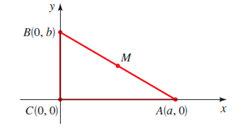# To verify: The point M is equidistant from the vertices of triangle ABC.### Precalculus: Mathematics for Calcu...

6th Edition
Stewart + 5 others
Publisher: Cengage Learning
ISBN: 9780840068071### Precalculus: Mathematics for Calcu...

6th Edition
Stewart + 5 others
Publisher: Cengage Learning
ISBN: 9780840068071

#### Solutions

Chapter 1.8, Problem 48E
To determine

## To verify: The point M is equidistant from the vertices of triangle ABC.

Expert Solution

### Explanation of Solution

Given information:

The point M is the midpoint of the line segment AB and figure,Formula used:

Mid-point formula between two points X(a1,b1) and Y(a2,b2) is mathematically expressed as,

(a1+a22,b1+b22)

Distance formula between two points X(a1,b1) and Y(a2,b2) is calculated as,

d(XY)=(a2a1)2+(b2b1)2

Proof:

Consider the given figure,In the above figure, M is the mid-point of the line segment AB.

Recall the mid-point formula between two points X(a1,b1) and Y(a2,b2) is mathematically expressed as,

(a1+a22,b1+b22)

So, coordinates of point M will be calculated as,

(a+02,0+b2)=(a2,b2)

Now, to show that M is equidistant from the vertices of triangle ABC, prove that the distance from point M to the vertices are equal, i.e.,

d(A,M)=d(B,M)=d(C,M)

Recall the distance formula between two points X(a1,b1) and Y(a2,b2) is calculated as,

d(XY)=(a2a1)2+(b2b1)2

So, distance between A and M is calculated as,

d(A,M)=(a2a)2+(b20)2=a24+b24=a2+b24=a2+b22

Distance between B and M is calculated as,

d(B,M)=(a20)2+(b2b)2=a24+b24=a2+b24=a2+b22

Distance between C and M is calculated as,

d(C,M)=(a20)2+(b20)2=a24+b24=a2+b24=a2+b22

Since, d(A,M)=d(B,M)=d(C,M) , so, it is proved that M is equidistant from the vertices of triangle ABC.

Thus, it is proved that point M is equidistant from the vertices of triangle ABC.

### Have a homework question?

Subscribe to bartleby learn! Ask subject matter experts 30 homework questions each month. Plus, you’ll have access to millions of step-by-step textbook answers!